# Sequences on the GMAT

Understand how to handle these tricky upper level Quant problems!

## Definitions

A sequence is a list of numbers that follow some mathematical patterns.  More formally, a sequence is a function whose inputs are limited to the positive integers.  Terms are denoted by a letter for the whole sequence, and in the subscript, the index, which is the place on the list.  Here are some examples of common sequences.  First, (A) and (B) are arithmetic sequences:

A)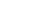,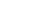,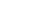,,, …

B)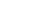,,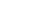,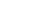,, …

In an arithmetic sequence, the same “common difference” is added or subtracted each time.  In (A), we add 3 to each term to get the next.  In (B), we subtract 4 to get each new term.  If you graphed the terms of an arithmetic sequence against the indices, the dots would follow a straight line in the x-y plane.

The next two, sequences (C) and (D), are geometric sequences:

C),,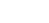,,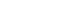, …

D),,,,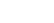, …

In a geometric sequence, the same “common ratio” is multiplied or divided each time.  In (C), we multiply each term by 3 to get the next.  In (D), we divide by 2 to get each new term.  If you graphed the terms of an geometric sequence against the indices, the dots would follow an exponential function in the x-y plane.

Notice also — (D) begins with a “zeroth” term.  The first term of a sequence can have an index of either 1 or 0: both appear on the GMAT.

There are other exotic sequences in mathematics.  Here are two more

E)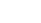,,,,,, …

F),,,,,,  …

Both of these are famous in mathematics.  The first is the Fibonacci sequence, which I will discuss further below.  The second, known in math as the Partition Function (http://en.wikipedia.org/wiki/Partition_(number_theory)) gets into way way more difficult math that you need to know for the GMAT.

## Explicit Series

A explicit series is a series in which the general rule for finding each term can be stated, either verbally or mathematically.  Sometimes the GMAT will give you the general rule for a sequence in algebraic form: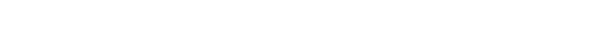(A) 4

(B) 6

(C) 8

(D) 12

(E) 18

If the rule is given algebraically, all we have to do is plug in the index number to find the value of each term.  Here, we plug in n = 3 to find the third term, and plug in n = 4 to find the fourth term.  The third and fourth terms are: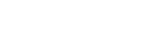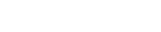so the difference between them is 12 – 6 = 6, which is answer B.  I will give another practice question of this genre at the end.

The explicit description might also be given verbal.  For example

2) In each term of a sequence, 9 is added to get the next term.  If the first term is 2, what is the eighty-first term?

(A) 632

(B) 695

(C) 713

(D) 722

(E) 731

Notice, the rule for the whole sequence is articulated: we know it is an arithmetic sequence with a common difference of 9.  You can try this question now.  I will show the solution at the end of this article.

## Recursive Sequences

In mathematics, a recursive definition is one where you need the result at end of each step in order to proceed to the next step.  A sequence has a recursive definition if the only way to calculate the third term is first to calculate the second term, and the only way to calculate the fourth term is first to calculate the third term, etc. etc.  Clearly, if we were asked for, say, the sixth term of a recursive sequence, we would have to calculate each and every term along the way: there’s no “shortcut” we can use to shoot directly to the sixth term.

Recursive defined sequence on the GMAT are almost always given in algebraic form.  Here’s an example of a question involving one.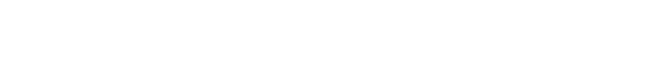(A) 4

(B) 11

(C) 20

(D) 31

(E) 116

Whenever you see the nth term equal to some expression involving an (n – 1)th term, that’s a recursively defined sequence.  The idea is: to find the n = 3 term, you would have to plug the n – 1 = 2 term into the formula; to find the n = 4 term, you would have to plug the n – 1 = 3 term into the formula; etc.  I will discuss a full solution to this one below.

Sometimes, a sequence is recursively defined not simply in terms of the previous term, but in terms of the previous two terms.  The most famous sequence defined this way is the Fibonacci Sequence:

1, 1, 2, 3, 5, 8, 13, 21, 34, 55, 89, 144, …

This is famous because these numbers are found all over Nature: the number of seeds in almost any fruit, the number of petals on any flower, even the number of digits on one human hand or foot.  Some theorists feel Fibonacci Numbers even give insight into the fluctuations of the stock market: http://www.goldennumber.net/fibonacci-stock-market-analysis/

The Fibonacci series has a recursive definition that can be written algebraically as: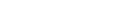Think about what that says.  If I want the n = 5 term, for example, I have to take the sum of the n – 1 = 4 term with the n – 2 = 3 term.  To find any term, I have to add the two previous terms.  You start with two 1’s, follow that rule, and you generate the Fibonacci sequence.

Whenever you see the nth term expressed as some combination of the (n-1)th and (n-2)th terms, that’s a recursively defined sequence in which each term is defined in terms of the previous two terms.  You will always be given two “starter” terms to such a sequence.  Here’s a sample problem involving one.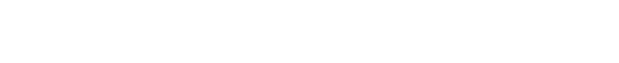(A) 1

(B) 7

(C) 22

(D) 155

(E) 721

I will give a complete solution to this below.

If you understand how to plug into the algebraic sequences, and how to extend the patterns and find terms of the verbal defined sequences, you will be able to handle everything the GMAT throws at you involving sequence.

## One Further Practice Question

As promised, another question of the first type discussed.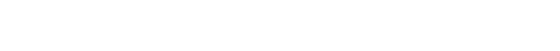(A) 1/2

(B) 2/7

(C) 5/8

(D) 7/10

(E) 7/12

## Practice Question Explanations

Practice question #1 was explained in the text of the article, immediately following the question, and the answer (B) was stated there.

2) Let’s think about the first few terms of this sequence.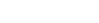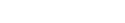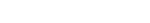To find each new term, we add 9. The result is, each term equals 2 plus a sum of 9’s.  How many nines?  Well, the second term has one 9, the third term has two 9’s, the fourth term has three 9’s, so it’s clear the nth term would have a sum of (n – 1) 9’s.  Thus, the eighty-first term would be 2 plus eighty 9’s.  Well, eighty 9’s = 80*9 = 720, so the eighty-first term = 722, answer D.

3) This is a recursively defined sequence, so we have to find the values of each term up until the one we want, the 5th term.  Fortunately, the “starter” term we are given is the 2nd term, so we have a bit of a head start.  We don’t know why the question started us with the second term, instead of the first, term, but it works to our advantage, so we won’t complain.  To find the next term, use the recursion formula: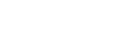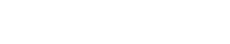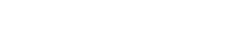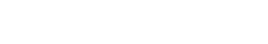Notice, there’s no shortcut with recursive sequences: we have to find the third term = 4 and then use that to find the fourth term = 11, then used that to find the fifth term = 116.  There’s no way to go directly to the fifth term with calculating each one of the previous terms.  The fifth term = 116, answer E.

4) This is a recursively defined sequence, defined in terms of the previous two terms, so we have to find the values of each term up until the one we want, the 6th term.  Again, we have to find the terms one by one, using the recursion formula.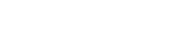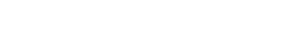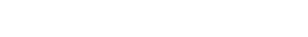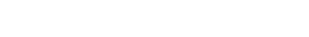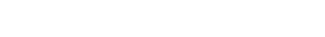So, the sixth term = 22, answer = C.

5) Ah, an explicitly defined sequence!  After recursive sequences, this is much easier.  All we have to do to find the 7th term is to plug n = 7 into the formula.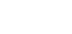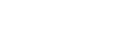The seventh term is 1/2, Answer = A.

## Even More Practice Questions

#### Special Note:

To find out where sequences sit in the “big picture” of GMAT Quant, and what other Quant concepts you should study, check out our post entitled:

What Kind of Math is on the GMAT? Breakdown of Quant Concepts by Frequency

## Author

•Mike served as a GMAT Expert at Magoosh, helping create hundreds of lesson videos and practice questions to help guide GMAT students to success. He was also featured as "member of the month" for over two years at GMAT Club. Mike holds an A.B. in Physics (graduating magna cum laude) and an M.T.S. in Religions of the World, both from Harvard. Beyond standardized testing, Mike has over 20 years of both private and public high school teaching experience specializing in math and physics. In his free time, Mike likes smashing foosballs into orbit, and despite having no obvious cranial deficiency, he insists on rooting for the NY Mets. Learn more about the GMAT through Mike's Youtube video explanations and resources like What is a Good GMAT Score? and the GMAT Diagnostic Test.